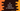# C# program to convert Celsius to Fahrenheit## C# program to convert Celsius to Fahrenheit:

This C# program will show you how to convert a celsius value to fahrenheit. The program will take the celsius as input from the user and print out the fahrenheit value.

With this program, you will learn how to read user input values and how to do mathematical calculations in C sharp.

### Formula to convert celsius to Fahrenheit:

We can use the below formula to convert a celsius value to Fahrenheit:

``Fahrenheit = (Celsius * 9)/5 + 32``

We can read the value of Celsius and print the Fahrenheit using it.

### C# program:

Below is the complete C# program that reads the Celsius value from the user and prints the Fahrenheit conversion:

``````using System;

public class Program
{
public static void Main()
{
double fahrenheit, celsius;

Console.WriteLine("Enter the Celsius value : ");

fahrenheit = (celsius * 9)/5 + 32;

Console.WriteLine("Fahrenheit : {0}",fahrenheit);

}
}``````

Here,

• We created two double variables fahrenheit and celsius to hold the fahrenheit and ceisius value.
• Then we are taking the celsius value as input from the user and calculating the fahrenheit using the above formula.
• Finally, we are printing the fahrenheit value on the console.

It will give output as like below:

``````Enter the Celsius value :
100
Fahrenheit : 212``````